# Wavelet Scattering explanation?

How does Wavelet Scattering work, intuitively? What are its motivations, and how's it differ from the (continuous) Wavelet Transform? Can it be visualized?

Wavelet Scattering is an equivalent deep convolutional network, formed by cascade of wavelets, modulus nonlinearities, and lowpass filters. It yields representations that are time-shift invariant, robust to noise, and stable against time-warping deformations - proving useful in many classification tasks and attaining SOTA on limited datasets.

Core results and intuition are provided in this answer, advanced details in another. Continuous Wavelet Transform is a necessary requisite; I recommend this tutorial. Answer covers 1D scattering, but much generalizes to images; for a description covering both, see this lecture.

### Continuous Wavelet Transform

As a short review, CWT measures similarity of the signal with wavelets of varying frequency and scale, at each point in time. We can visualize as follows, taking CWT of exponential chirp: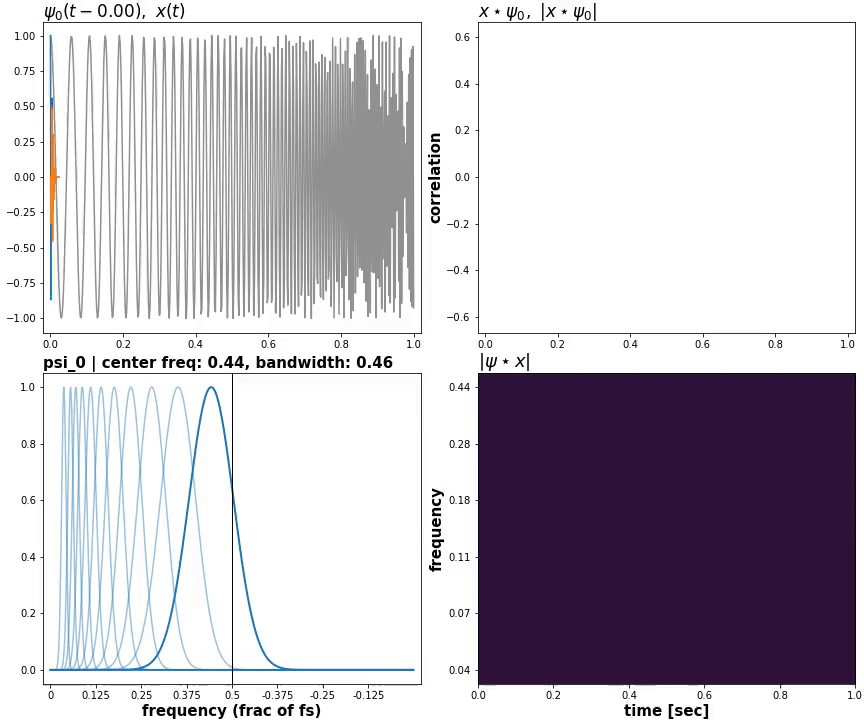1. Slide wavelet psi_i by some time shift tau
2. Compute similarity (inner product, sum(x * psi))
3. Repeat for all tau and psi_i

### Scattering (first-order)

Is modulus of CWT convolved with lowpass, "temporal averaging":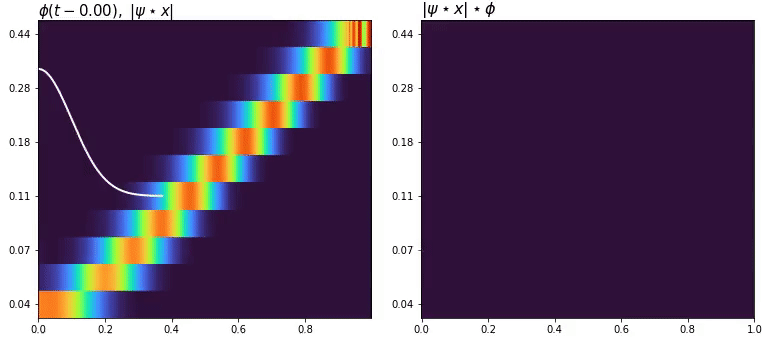This averaging imposes 1) time-shift invariance, and 2) stability against time-warping deformations. The overall operation is

$$S_1 x(t, \lambda_1) = |x \star \psi_{\lambda_1}| \star \phi \tag{1}$$

yielding first-order scattering coefficients. $$\lambda_1$$ is center frequency of first-order wavelets (indexed by i in the gif). The convolution is of the lowpass filter with every individual row of CWT, Sx[i] = conv(phi, CWT[i]).

### Second-order

Proceeds by convolving modulus of CWT with second-order wavelets:

$$W_2 x(t, \lambda_1, \lambda_2) = |x \star \psi_{\lambda_1}| \star \psi_{\lambda_2} \tag{2}$$

This "second CWT" is done directly atop modulus of first CWT - that is, a second-order wavelet takes the entire first CWT as input, convolving with every row, just like the lowpass.

Second-order coefficients are obtained by convolving modulus with lowpass:

$$S_2 x(t, \lambda_1, \lambda_2) = ||x \star \psi_{\lambda_1}| \star \psi_{\lambda_2}| \star \phi \tag{3}$$

and so on. Higher orders proceed the same, convolving wavelets with modulus of previous order, followed by modulus and lowpassing:

$$S_m x(t, \lambda_1, ..., \lambda_m) = |||x \star \psi_{\lambda_1}| \star ... | \star \psi_{\lambda_m} | \star \phi \tag{4}$$

### Notation

$$Wx = x \star \psi;\ \ Ux = |Wx|;\ \ Sx = Ux \star \phi$$

$$W$$ for wavelet transform, $$U$$ for unaveraged coefficients (after modulus), $$S$$ for scattering (averaged coefficients).

OA = elaborated in other answer

### Invariance, information preservation

Why higher orders? The idea is to recover information lost in previous orders.

Modulus

• is a nonlinearity, without which stacked convolutions are equivalent to one convolution
• extracts amplitude modulations
• enables extracting higher-order modulations
• enables averaging that imposes useful invariants
• but loses phase (some of it; see below)

Averaging

• imposes time-shift invariance
• improves stability against time-warping deformations
• but loses time localization

Second order recovers information lost by averaging by convolving with unaveraged coefficients:

$$W_2x = U_1x \star \psi \tag{5}$$

A proper wavelet transform is perfectly invertible, thus all information contained in $$U_1x$$ is carried to $$W_2x$$. Note this does not recover phase, since it was lost at $$U_1x$$ (we'd have to operate on $$W_1x$$ instead). However, most of phase information is encoded in redundancy of the transform; a scalogram is strongly invertible, ~perfectly for some signals (OA).

### Analysis vs Synthesis

Analysis information is the very goal of transforming data in the first place: if all we cared for was preserving the input (synthesis information), simply don't do a transform. In context, analysis information includes:

1. frequency and amplitude localization and modulation rates
2. latent linearity (OA)
3. sparsity, stability, coherence, and robustness of representation

Averaging serves 3, modulus and higher orders serve 1 and 2. Synthesis remains nonetheless a significant consideration, since if synthesis information is lost, virtually all other forms of information are lost. Scattering is invertible, to an extent (OA).

In practice, scattering coefficients are subsampled, without losing information (it's lost at averaging). For intuition, note that STFT with hop_size > 1 is aliased, which loses analysis (but not necessarily synthesis) information. Regardless it's widely used, with hop_size in hundreds and thousands. That's because a sparse and redundant representation like STFT/CWT loses much less information in analysis per loss in synthesis: output can be smaller than input, yet more "valuable".

For classification, the idea is to balance inter-class variability against intra-class invariability: want coefficients that differ for different classes, but not for same. It's exactly what scattering strives for.

### Fourier failure

The Fourier Transform is an orthogonal representation: while it packs the most synthesis information per sample, it bears minimal analysis information. It's brittle against noise and deformation, and changes significantly with changes to input that are meaningless for a given task. Elaborated in References.

CWT decomposes a signal using a redundant "dictionary" of time-frequency atoms. In language, a rich dictionary contains many words, and some words will have many meanings, which allows expressing a complex idea in a short sentence (borrowing analogy from S. Mallat). Likewise, a rich dictionary of wavelets enables superior sparsity and 'expressivity'.

### vs MFCC

MFCC also averages, its own way, but loses information; this limits its effectiveness in many applications, which require larger-scale invariants. MFCC is also much less rich in analysis information and configurable invariants per lacking higher-order extensions.

### Time-shift invariance

Consider a windowed sine shifted in time, and its CWT:The sine shifts, and CWT shifts by same amount (this is "translation equivariance", sometimes referred to as invariance; it's invariant in the sense of features (e.g. shape, energy) not changing).

Second row shows scattering with different $$T$$, the scales of invariance. It's invariance in two senses:

1. Euclidean distance bewteen coefficients is less for greater $$T$$.
2. Variability across a duration lesser than $$T$$ is killed$${}^*$$. (Greater $$T$$ means greater lossless subsampling, meaning fewer samples represent the same variation. So x16 subsampling means, pre-subsampling, 16 samples don't represent any variation that 1 sample can't)

1 is better illustrated by comparing the shifts directly; taking max row of each:Plotting (relative) distance between full representations, $$||S_1(x(t)) - S_1(x(t - \tau))|| / ||S_1(x(t))||$$, vs $$T$$, where $$||\cdot|| = \sqrt{\sum{|\cdot|^2}}$$:Shrinks as expected, with convergence to $$T = 1\text{ sec}$$ having ~zero distance (global average pooling).

$$*$$: it's "killed" on each individual coefficient, rather than on the signal itself. Variations on scales $$ is preserved partly via the redundancy of the transform, and recovered fully with higher orders of scattering, so only sense $$1$$ is applicable to the overall representation.

### Time-warp stability

A time warp is a transformation

$$x(t) \rightarrow x(t - \tau(t)),$$

where $$|\tau'(t)| < 1$$ ($$=1$$ implies time stopping, and $$>1$$ time reversal. $$=0$$ is a pure shift and is included). Suppose the simplest case of $$\tau = \epsilon t$$ upon a sine, i.e. $$x((1 - \epsilon)t)$$, and compare CWT & STFT:STFT partials shift more at higher frequencies for same time warp, eventually not overlapping the original in frequency at all. Despite $$\epsilon$$ being small, STFT suggests formation of an entirely new partial - and partials aren't shifted proportional to $$\epsilon$$. Meanwhile, CWT partials overlap at all frequencies, and by same amounts for sufficiently small $$\epsilon$$, and in proportion to $$\epsilon$$. Example with non-constant $$\tau'$$:Same warp distorts STFT more severely at higher frequencies, while CWT the same. CWT is equivariant to multiplicative time-warps, and approx. to small additive time-warps (OA).

Next we apply the same warp at varying intensities: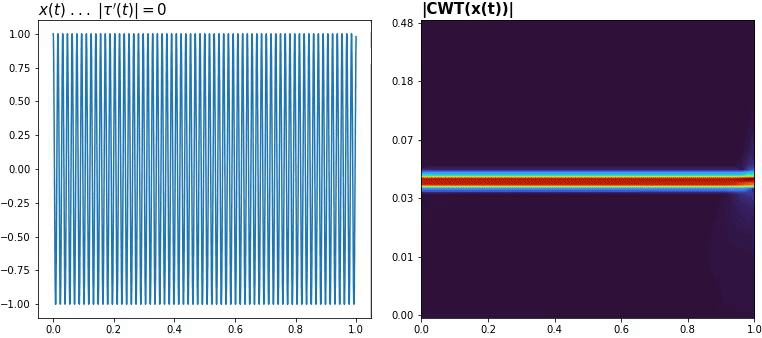Warps near $$\max |\tau'| = 1$$ are severe (and shouldn't be ignored), but seem manageable when small. Warping works same for nonstationary signals - e.g. amplitude-modulated exponential chirp: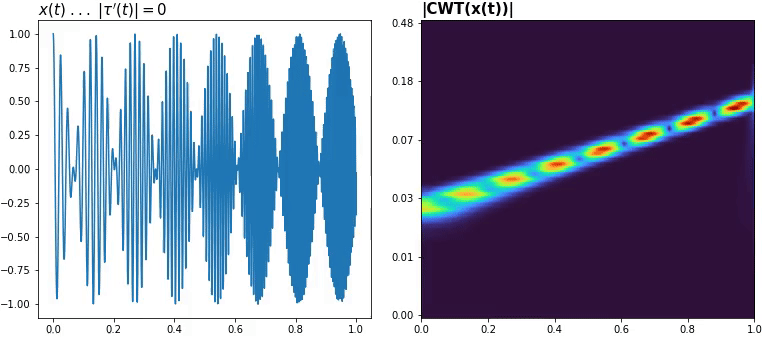Show all warps simultaneously via heatmap:One can show that scalogram temporal averaging has a frequential averaging effect on spectrogram (Ref 1 Sec 2); intuitively, time averaging dilates coefficients in time, overlapping otherwise separate bands.

Scattering a reasonable time warp for all $$T$$: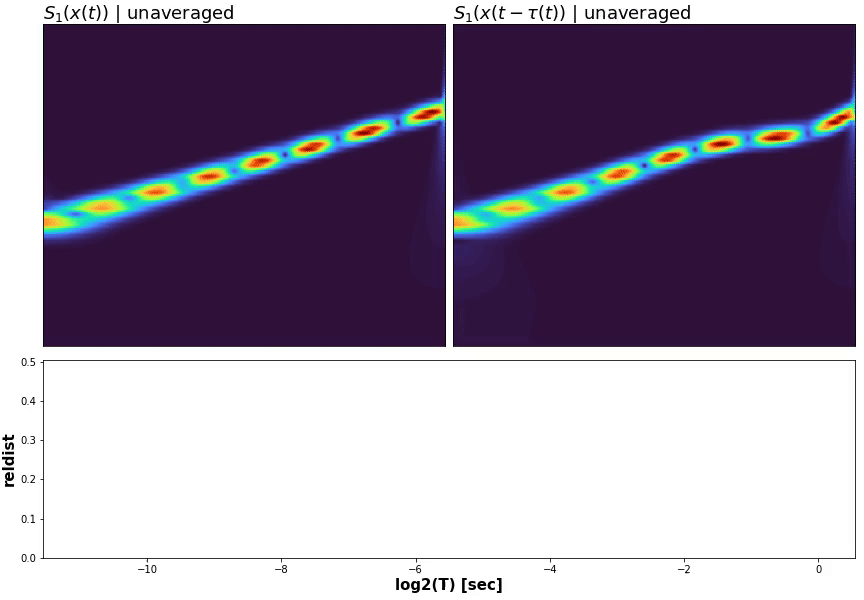Distance between warped and unwarped is reduced as expected.

To illustrate stability: keep $$T$$ fixed, increase size of deformation. If coefficient distance grows with size of deformation, then we're stable: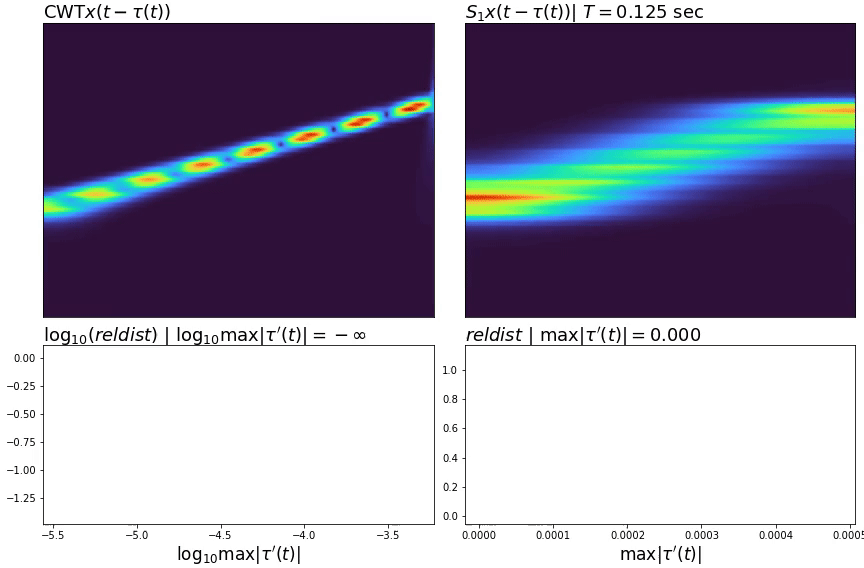Both CWT and scattering exhibit stability, but scattering's distance is globally lower. This owes to CWT's scale equivariance (OA), and the warp deforming mainly along frequency. Regardless, CWT isn't stable against more general warps; to show an extreme, consider time-shifting (a form of warping) a high frequency pulse: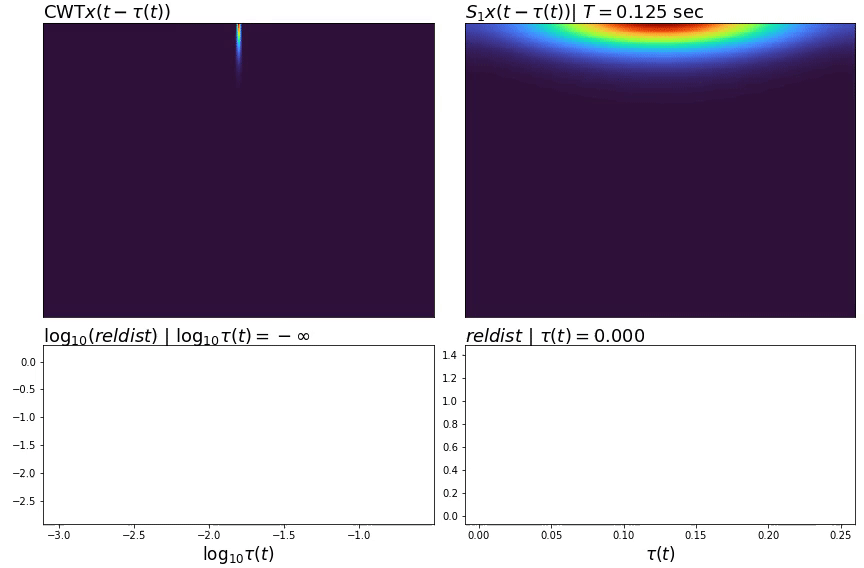Scattering's distance grows perfectly with timeshift size, while CWT unoverlaps completely early on. Note stability persists beyond the scale of invariance. For further detail see OA, "Invariance" vs "Stability".

### Why shift invariance?

Useful when we care for what happens, not when, or the fine aspects of how. The order of events may be preserved, but blended together. E.g:

• If we increase scale of invariance, we may tell that words are spoken, but not what the words are, nor possibly their place in sentence. If we lower, we may capture expression in pronouncing individual letters, but confuse which word (or letter) is spoken due to noise
• Few seconds of temporal context often suffice to recognize a speaker or musical instrument, but 30 might be needed to distinguish acoustic scenes which share some semantic context, e.g. a train from a subway station or a quiet street from a park (Ref 4 paraphrased).
• Without averaging, noise aside, there's too much variability (irrelevant stimuli) and a learner won't effectively separate classes.

The motivation for giving up any information at all, of course, is robustness to noise and diminished irrelevant stimuli: reducing intra-class variability while preserving inter-class.

The tradeoff is controlled by $$T$$. The higher the $$T$$, the more information lost; the higher the scattering order, the more information is recovered. Latter can always compensate and recover ~all information lost to averaging (OA).

• ML: if feeding coefficients as feature vectors to a classifier, a larger scale (per producing fewer samples) yields fewer training instances at classifier stage, increasing variance of estimation and risk of overfitting. In contrast, a scale too low may yield many instances that are 'silent' or lack stimuli pertinent to the class, hence increase in bias and risk of underfitting (Ref 4 par.). At the same time, if feeding to e.g. CNNs, lower scale enables more information to be handled by a learnable feature extractor, which works better with enough data (improved bias-variance).

### Why warp stability & linearization?

• If we play a cassette x1.5 faster, it'll still say "whale oil beef hooked" - but if we play it x2.5, it might say something else
• We want a translator to work with people who may speak at different speeds
• "banana" echoed is still "banana", and a faster ambulance isn't a truck (Doppler effect)
• "7" in different handwritings (image warps)

Stability is hence useful any time a small change in the rate of the source process keeps our 'labels' unchanged. Or, for isolating a deformation from process of interest.

Linearizing permits approximating $$\Phi(x) - \Phi(x_\tau)$$ with a linear operator; deformations of this kind hence form a linear space, discriminable by a linear classifier. Invariants or covariants can be built in the transformed space. See OA, "Invariance" vs "Stability" for further detail.

### Why not scattering?

1. Need strong time or time-frequency localization (then I recommend synchrosqueezing)
2. Don't need time-shift invariance, warp stability and linearization, etc (OA "Properties summary")
3. Don't need interpretability
4. Have lots of data

While scattering provides powerful analysis information, it also loses nontrivial synthesis information unless opting for several orders (OA, "Energy analysis"). Put simply, "whatever you can do by hand, a neural net can do better". (Generalizing, whatever we can do with method X, there's likely a method Y that does it better. If X loses information, then Y can no longer "do it better" because "it" is partly gone forever - else, Y can invert X and do anything else).

Put differently, if scattering properties aren't useful for the task, other transforms can better utilize the same info.

### Why the name, "scattering"?

Paraphrasing V. Lostanlen:

Coined as a nod to quantum mechanics: from the reddish shade of a sunset to the glistering of a pearl, "scattering" is an umbrella term encompassing many microscopic phenomena. The commonality is, all involve a radiation of some kind as well as a maze of nonuniformities. For the transform, the "radiation" is a sound pressure wave, the "maze" is wavelets, and "nonuniformities" are modulus nonlinearities.

My preferred view is via energy analysis: scattering preserves input's energy. That is, coefficients' energies sum to input's. Equivalently, the input is decomposed. The decomposition is along "paths", e.g. $$x \rightarrow \psi_{\lambda_1=.3} \rightarrow \psi_{\lambda_2=.2}$$, and the paths follow a tree-like structure, branching off at each node. This "branching" is like opening a fist, with the arm being the "node".

If we pour a bucket of water on soil, it'll "scatter" along varying paths, with total water being what was in the bucket. A plant absorbing water is inverse scattering: water flows in opposite direction and metabolizes (used to synthesize).

### Bioplausibility

There is neurophysiological evidence to suggest the mamallian auditory system performs temporal integration of amplitude modulations via a physically equivalent modulation filterbank (T. Dau, et al, 1997, A. Sek, et al, 2003, A. King, et al, 2019), akin to first two (unaveraged) layers of scattering.

### Applications

List not exhaustive. Some attain SOTA on trialed datasets.

### Related material

1. Joint Time-Frequency Scattering: extends wavelet scattering, further increasing discriminability; covered here.
2. Scattering Invariant Deep Networks for Classification - lecture series, contrasting scattering w/ CNNs
3. Deep Scattering Spectrum - scattering properties, derivations, applications
5. "Center frequency" explanation - intuition on a concept central to time-frequency analysis

### References

1. Deep Scattering Spectrum - J. Anden, S. Mallat, 2013
2. Wavelet Tour - S. Mallat, 2008
3. Group Invariant Scattering - S. Mallat, 2012
4. Convolutional operators in the time-frequency domain - V. Lostanlen, 2017
5. Invariant Scattering Convolution Networks - J. Bruna, S. Mallat, 2012
6. Phase retrieval for the Cauchy wavelet transform - S. Mallat, I. Waldspurger, 2017
7. Exponential decay of scattering coefficients - I. Waldspurger, 2016

Other papers are also cited throughout the answers.